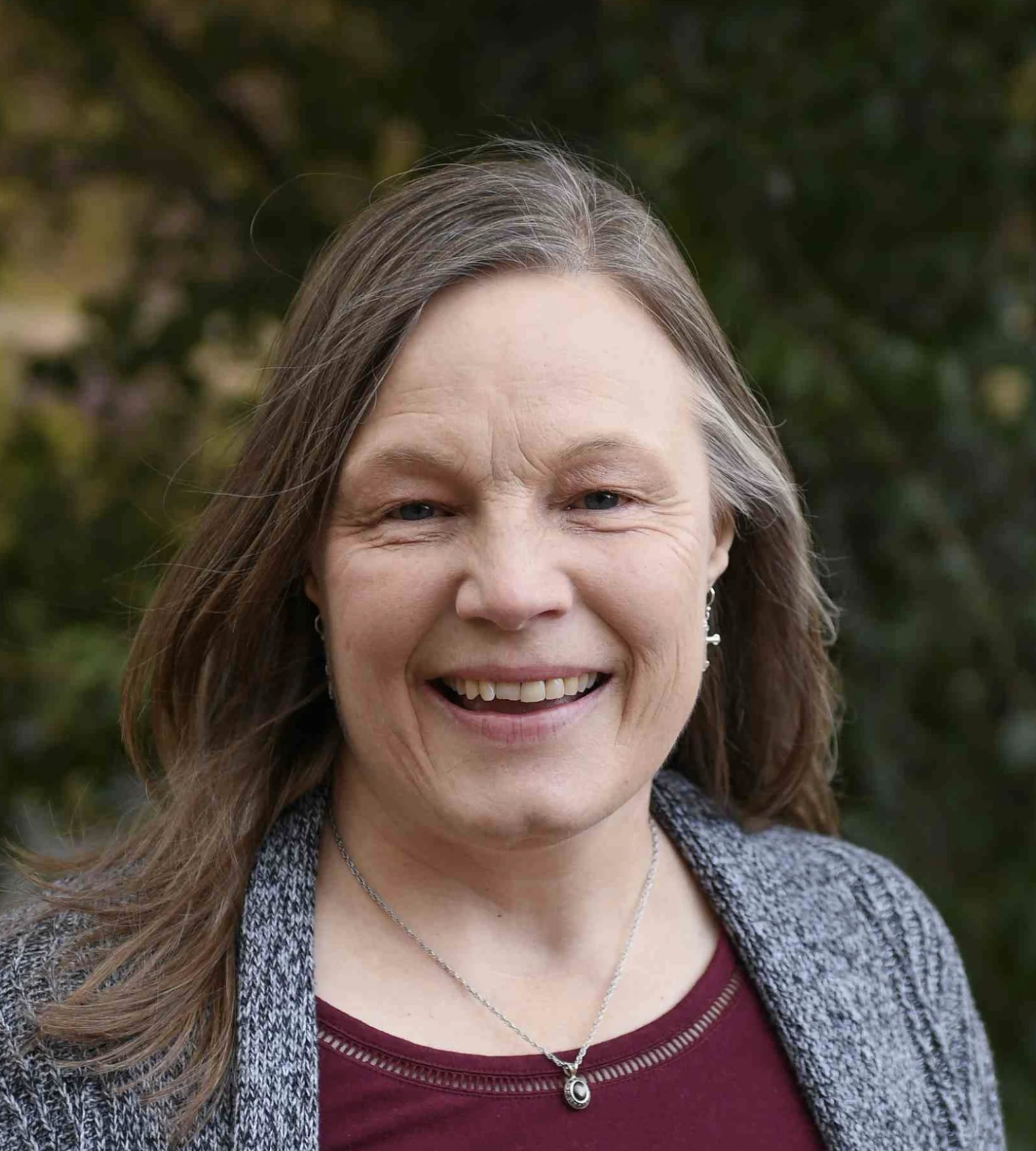# Pre-Algebra

\$500.00/year
Pre-Algebra
This class is currently archived, but if you're interested in it being taught again, you can express your interest here!
08/22/2022 - 05/12/2023
Full Year
1.0 credits in Mathematics

Taught by:Lori Miller

In the book Restoring All Things, Warren Cole Smith and John Stonestreet state, “…we glorify [God] by learning of Him and His world. To learn is an act of worship.” In the words of Galileo Galilei, “Mathematics is the language in which God has written the universe.” Learning mathematics is a powerful way to worship God.

Pre-Algebra is designed as a transition from arithmetic to algebra. It is a foundation for future courses in mathematics, science, and economics. In addition, algebraic thinking is a daily life skill. Thus, Pre-Algebra is designed to develop algebraic thinking, instead of merely acquiring techniques to solve problems. I would like to show students that mathematics is more than numbers and equations, that it encompasses logical, analytical, and creative thinking. I want to help them develop their problem-solving ability.

Topics covered will include properties of numbers, rational numbers, an introduction to graphs, functions, and sequences, exponents and roots, ratios and proportionality, percents, perimeter, area, volume, data and statistics, probability, equations and inequalities, graphing lines, and foundations of Geometry.

This 32-week course includes a weekly 1.5 hour recitation session. Students will be required to watch CTC videos and complete practice questions before class so they are prepared for in-class discussions and activities. Grades will be based on homework, class participation, unit tests, a final exam, and short oral presentations each semester.

### Course Objectives:

1. Students will recognize that mathematics is a beautiful God-given resource.
2. Students will learn to think algebraically.
3. Students will reinforce and expand skills learned in arithmetic and will begin to learn algebraic concepts in preparation for Algebra I.
4. Students will apply mathematical concepts to real life situations.
5. Students will discuss the mathematical concepts being learned.

### Texts:

• CTC Math online resource. Access provided through the instructor. Cost: \$30.00

## Course Files